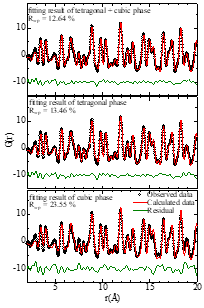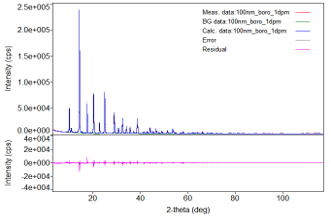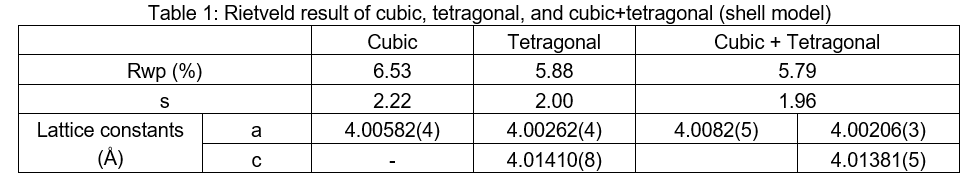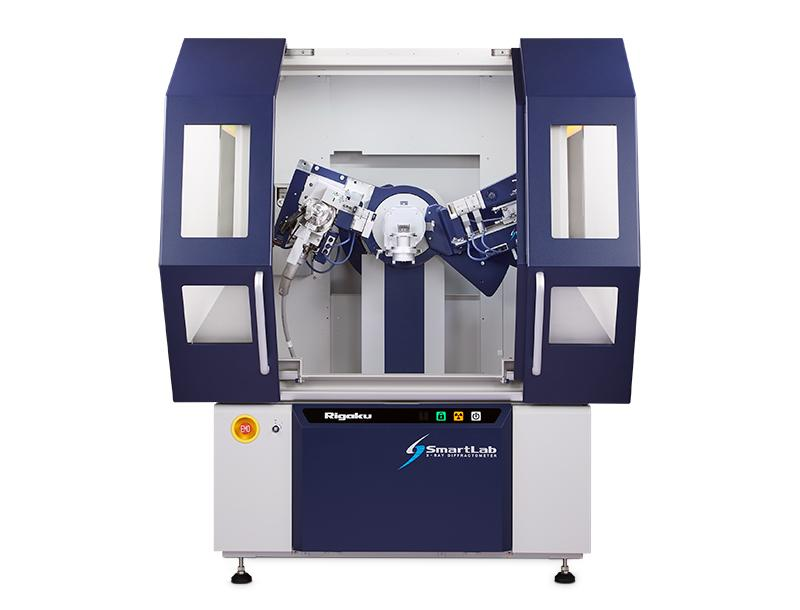# STRUCTURE DETERMINATION OF FERROELECTRIC NANO-POWDER BY PDF ANALYSIS

### Introduction

Ferroelectric BaTiO3 (BT) is widely used as a capacitor material. The ferroelectricity of BT is strongly related to its tetragonal crystal structure, but when the particle size becomes less than several tens of nanometers, the structure exhibits cubic symmetry and the ferroelectricity disappears. A structural model where the central core is tetragonal and the outer shell is made of a cubic crystal has been proposed for BT. In general, the crystal structure of powder materials is determined by Rietveld analysis of the X-ray diffraction profile. However, it has been reported that the tetragonality (i.e., the c/a ratio, where a and c are the lattice constants) of BT approaches 1 as the particle size decreases, making it difficult to determine whether the crystal structure is tetragonal and/or cubic by Rietveld analysis only. Recently, many researchers have utilized PDF analysis (atomic Pair Distribution Function, G (r)). Since G(r) provides information on the probability of atoms being located at a distance "r" from each other, even a crystalline material showing the smallest tetragonality can be analyzed considering the symmetry of the local structure. Here, we illustrate the results from an analysis of the structure of a nano-sized BT powder material using PDF analysis.

Measurements and Results

A φ=0.5 mm diameter boron-silicate capillary was filled with BT powder having an average particle size of about 30~50 nm. The sample was then measured by transmission X-ray diffraction. To measure the diffraction profile to a high Q range, we used a high-intensity Mo rotating anode source with a CBO-E focusing mirror designed for Mo radiation. The observed diffraction profile was corrected and normalized to obtain the structure factor S(Q), then Fourier transformed to G(r). Fig. 1(a) shows the G(r) plot and the calculated results using cubic, tetragonal, and cubic+tetragonal (shell) structural models, respectively. In the Rietveld results, the tetragonality of BT is close to 1 when simulated using a tetragonal model. In addition, the reliability factors (Rwp) from the three different models were almost the same (Table 1, Figure 1(b)). In the PDF analysis, Rwp decreased from 23.6% using a cubic model to 12.6 % when simulated by a cubic+tetragonal model. Therefore, it was concluded that the structure of the nano-sized BT powder is best described using a shell model combining cubic and tetragonal structures. These results indicate that PDF analysis is of great advantage in determining the crystal structure of powder materials with extremely small tetragonality or similar crystal structures.

(a)Fig. 1: (a) PDF results from nano-sized BT powder analyzed with cubic (bottom), tetragonal (middle), and cubic+tetragonal (shell) (top) models

(b)(b) Rietveld results using structural parameters obtained from the PDF results using the cubic+tetragonal modelRecommended equipment and software
► Automated multipurpose X-ray diffractometer SmartLab + Mo tube + Ellipsoidal multilayer X-ray mirror (CBO-E) + Integrated X-ray analysis software SmartLab Studio II (PDF plugin)## SmartLab